## 3.5 Exercises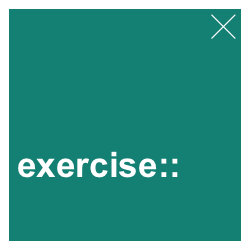### 3.5.1 Good vs. bad examples

Find and collect a good vs. a bad example of a plot (e.g., in brochures, newspapers, media reports, scientific articles, etc.).

1. What makes the good one good?
2. How could the bad one be improved?

Hint: There are many great sources for inspiration. For instance, check out r/dataisbeautiful at reddit.com.

### 3.5.2 Plot types

Evaluate and compare the following commands in R:

• plot(Nile)
• plot(cars)
• plot(iris)
• plot(Titanic)

Can you explain the types of the resulting plots in terms of the data provided?

#### Solution

The type of plot automatically chosen by R depends on the data provided to the plot() function (see Figure 3.4):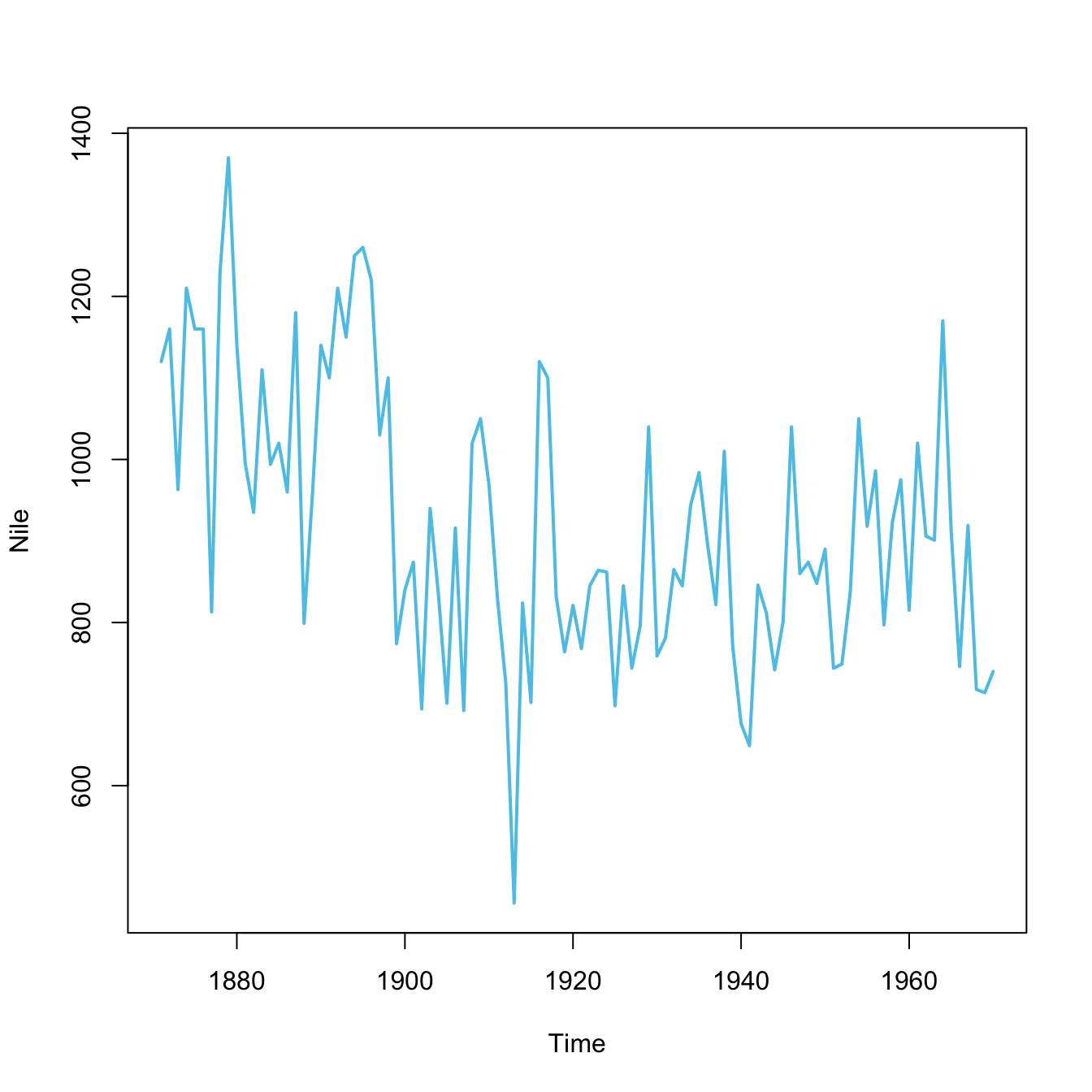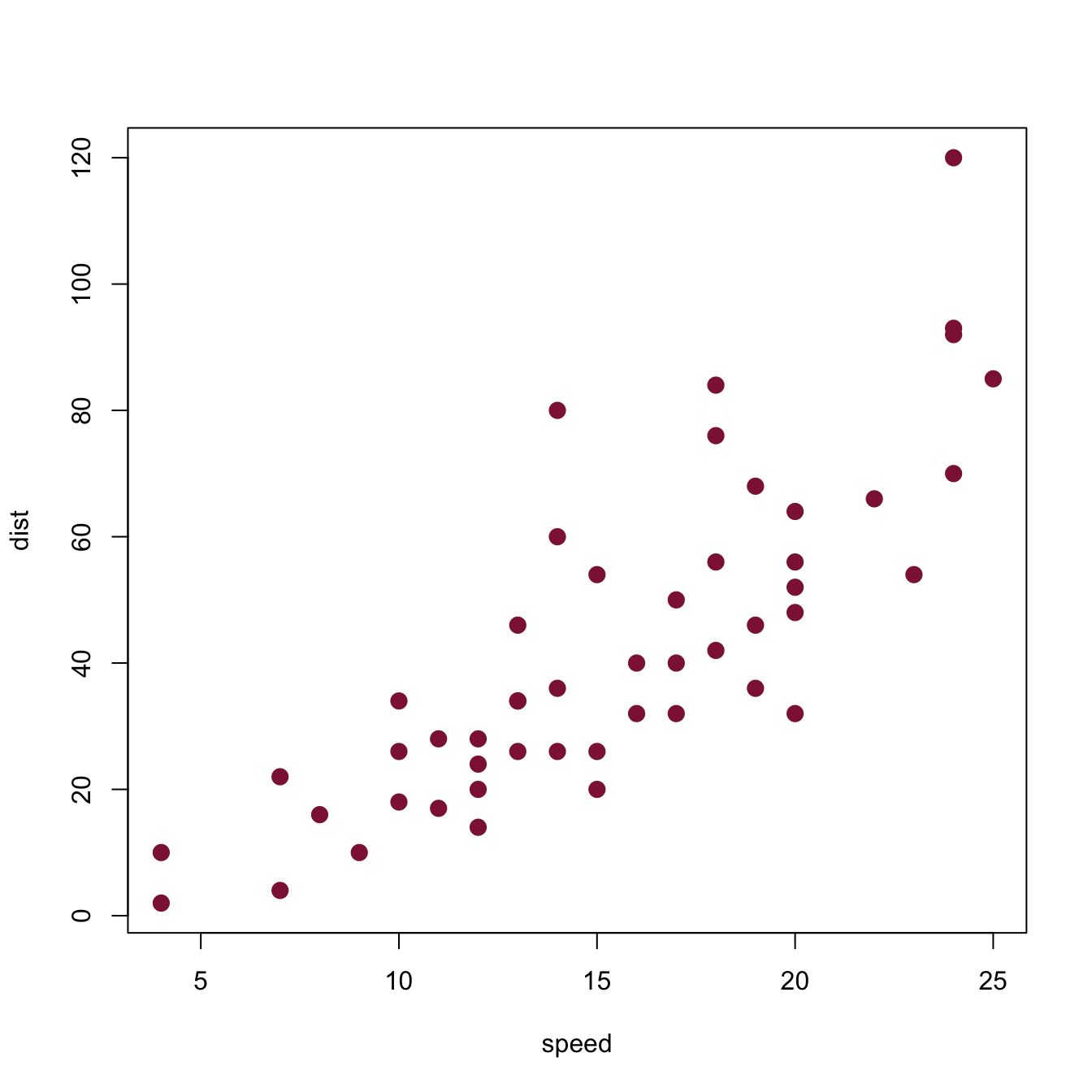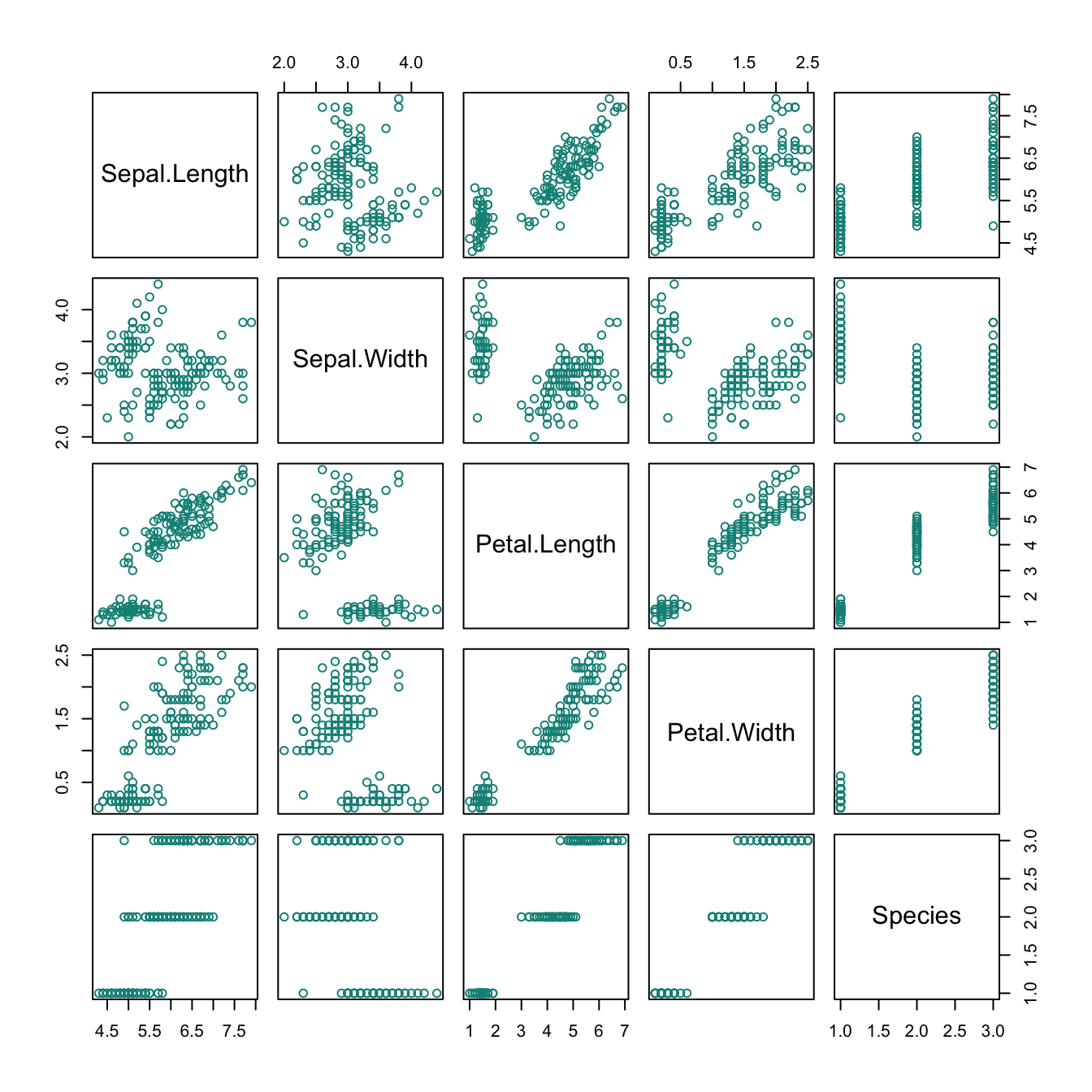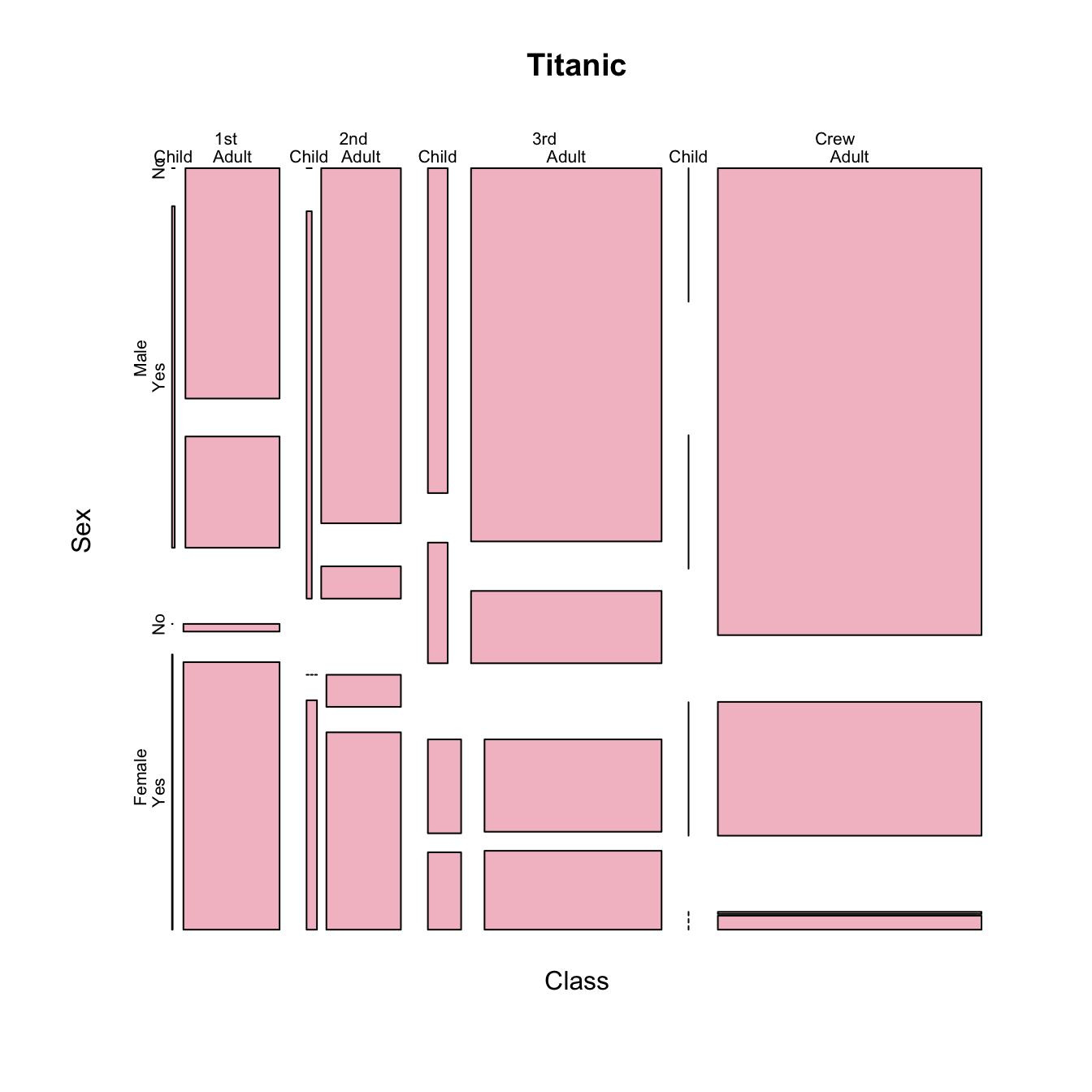Figure 3.4: Plots created by calling plot(x) with different types of objects x.

• plot(Nile) plots a time series as a line plot.
• plot(cars) plots a data frame of 50 observations and 2 variables as a scatterplot.
• plot(iris) plots a data frame with 150 cases and 5 variables (4 numeric, 1 character) as 5x5 scatterplots.
• plot(Titanic) plots the counts of 4 categorical variables as a (complex) mosaic plot.

### 3.5.3 Plotting the Nile

Plot the Nile data and justify your choice of plot.

#### Solution

Note that Nile data is a time series.

Figure 3.5 shows some options.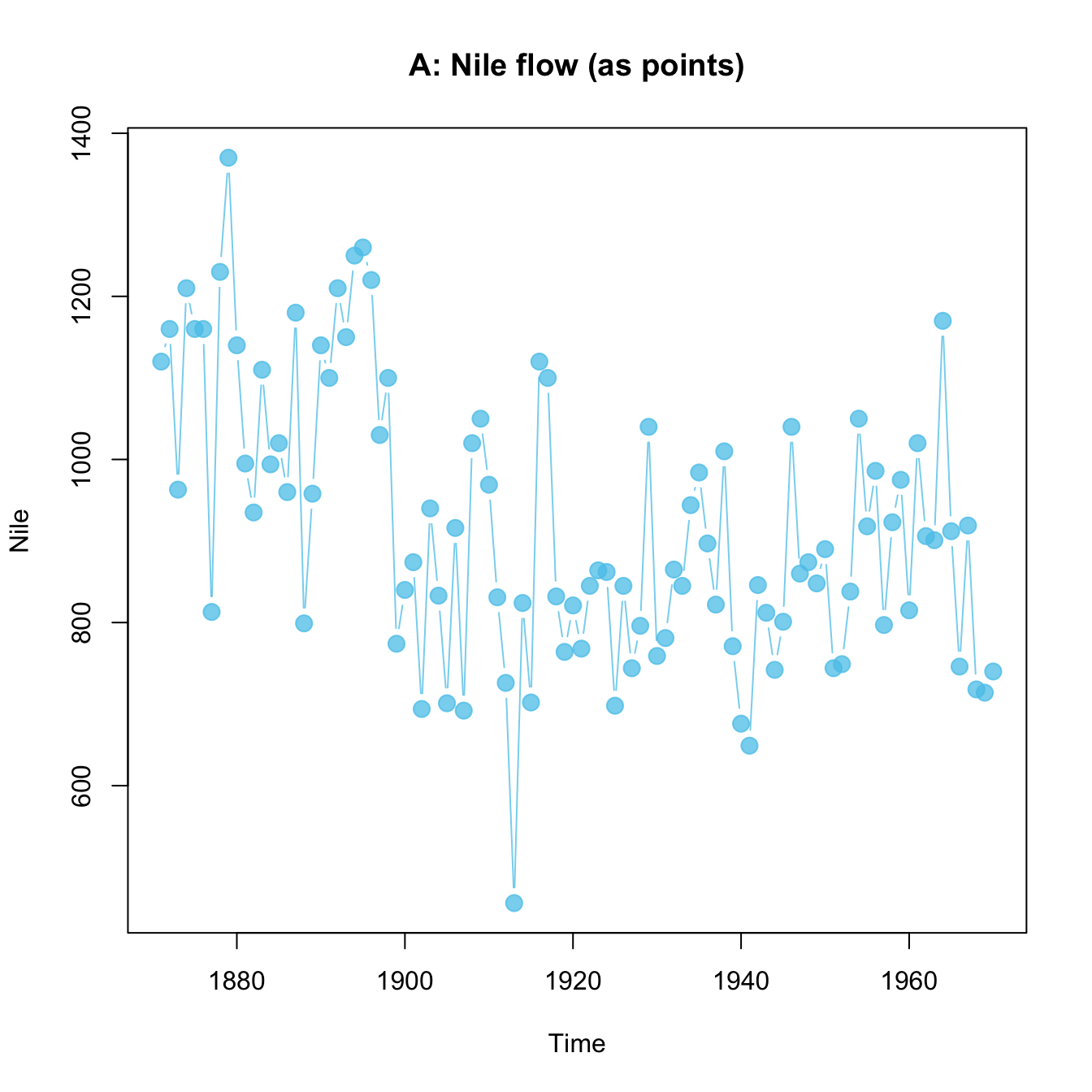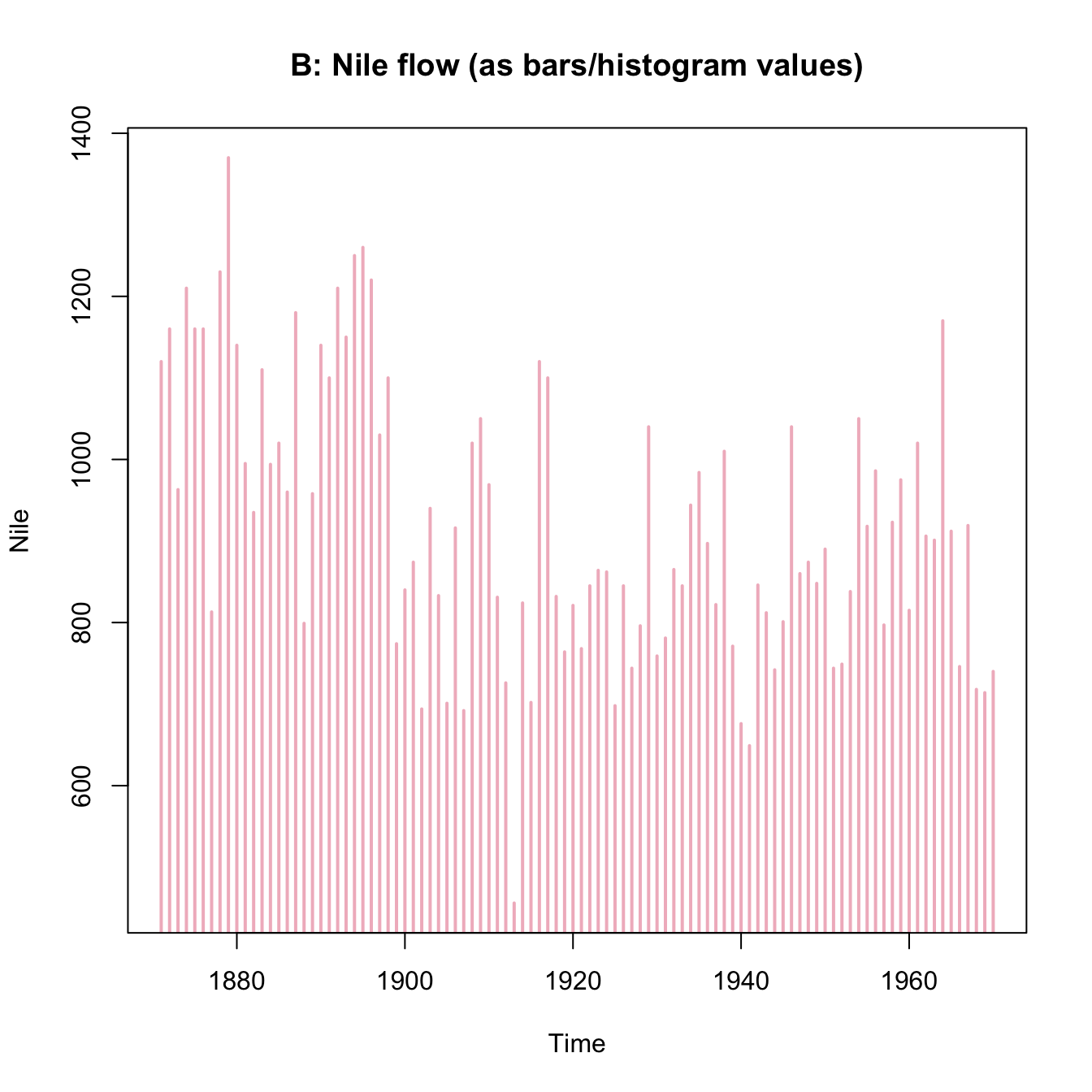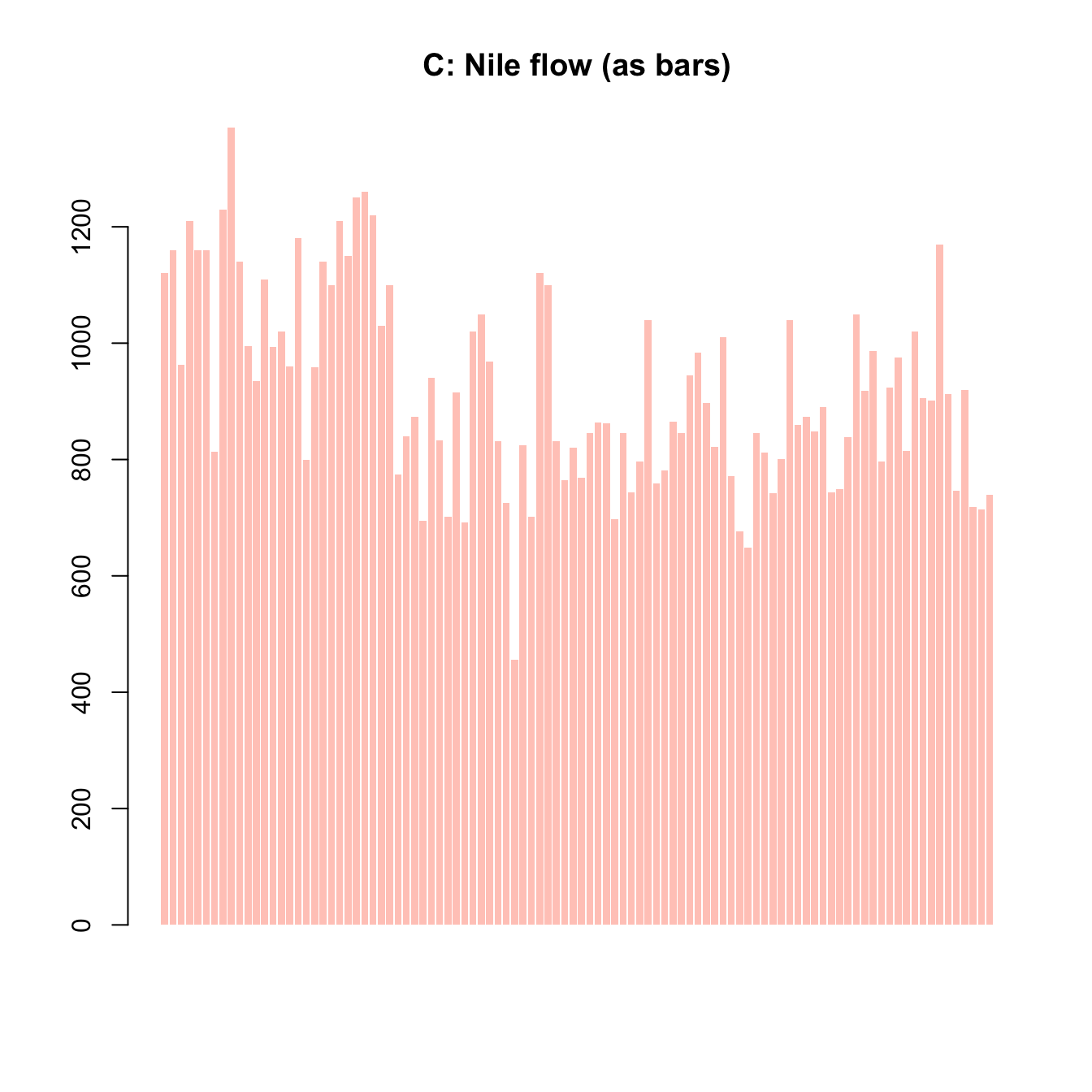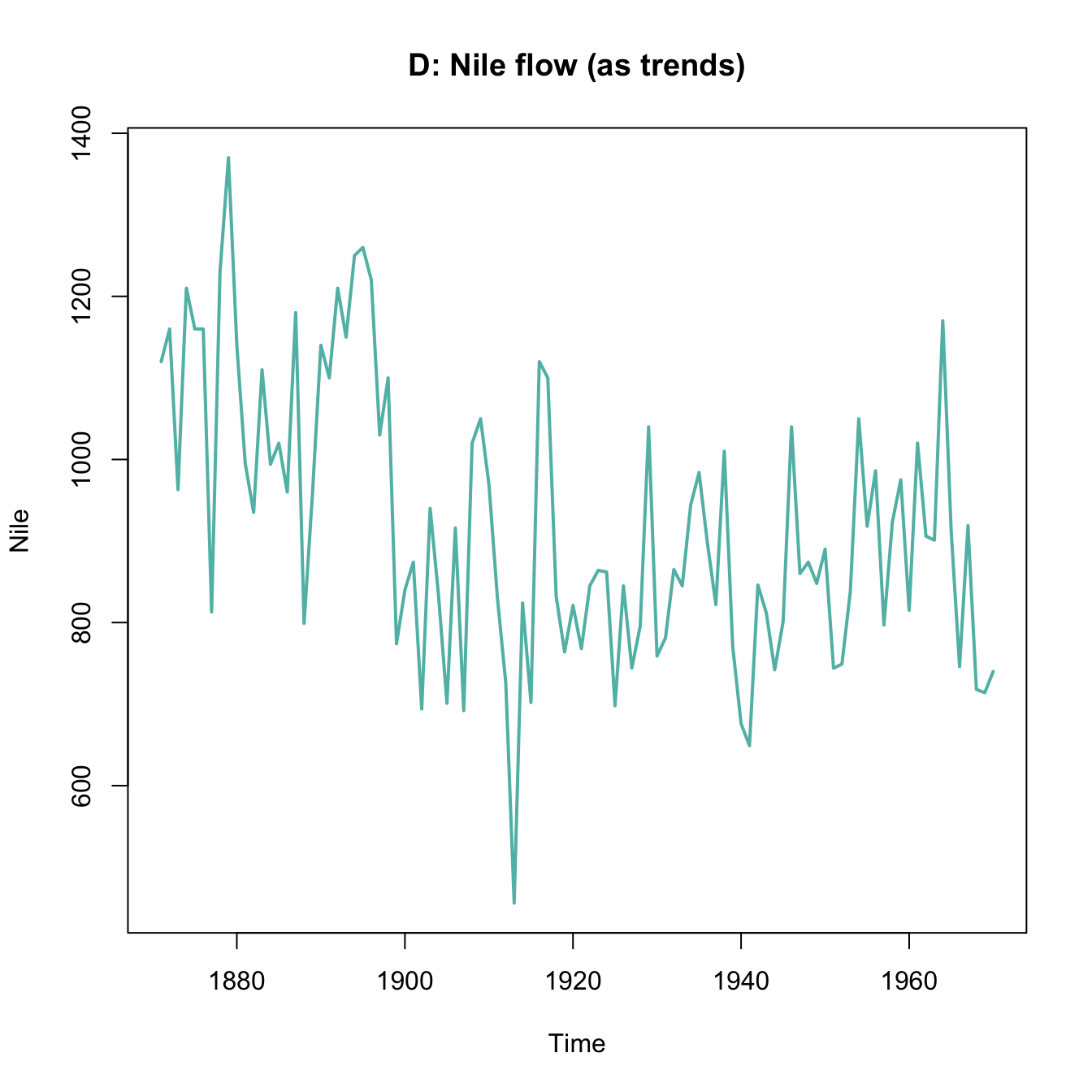Figure 3.5: Various ways of plotting the Nile data emphasizes different aspects.

### 3.5.4 Plotting a histogram

Using the mpg data from the ggplot2 package, create a histogram that shows the distribution of values of the cty variable (showing a car’s fuel consumption, in miles per gallon (MPG), in the city).

Getting the data:

mpg <- ggplot2::mpg

Before starting to plot anything, we should always first inspect and try to understand our data:

# Print data table:
mpg  # a tibble with 234 cars, 11 variables

# We are interested in the vector
mpg$cty # Note: ?mpg # describes the data #### Solution Here is how your histogram could look like: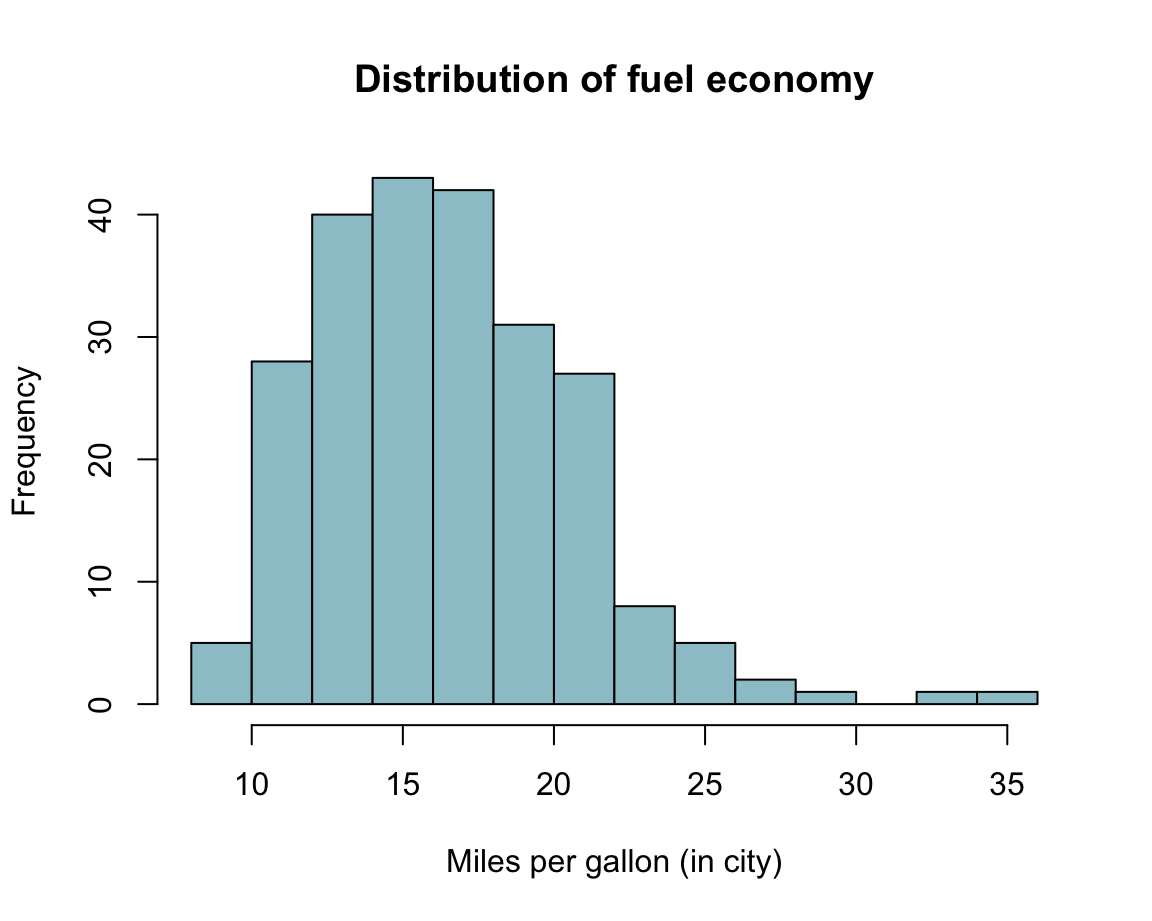### 3.5.5 Plotting a scatterplot Using variables from data: A typical scatterplot (using the mpg data from ggplot2): mpg <- ggplot2::mpg Create a scatterplot of this data that shows the relation between each car’s • x: engine displacement (i.e., variable displ of the mpg data), and • y: fuel consumption on highways (i.e., variable hwy of the mpg data). Can you avoid overplotting? #### Solution Here is how a solution could look like: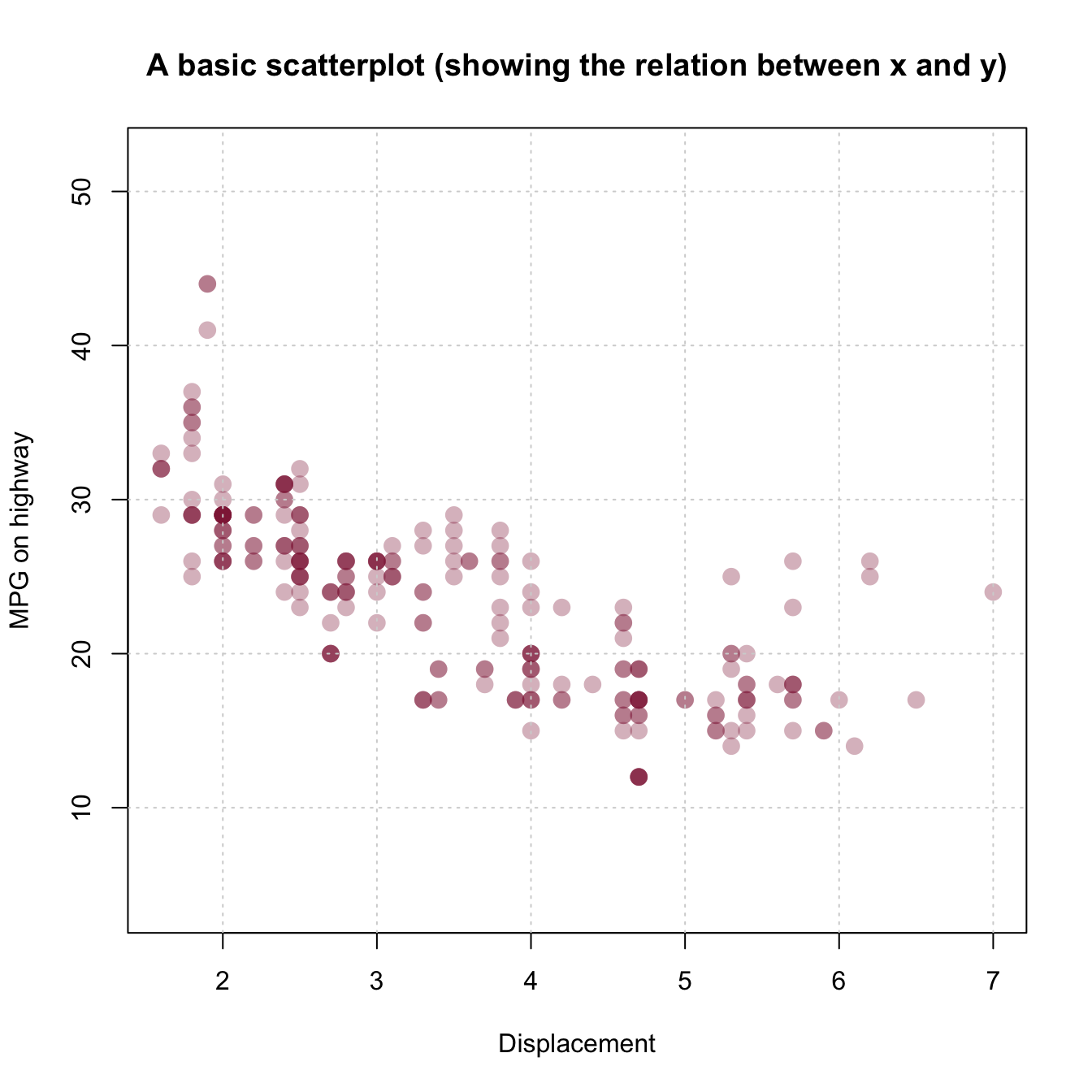### 3.5.6 Plotting a bar plot Re-create both bar plots of election data shown here with base R commands: 1. with stacked bars (i.e., one bar per year); 1. with bars beside each other (i.e., three bars per year). Here is the election data from of tibble de (and don’t worry if you don’t understand the commands used to generate the tibble at this point): library(tidyverse) ## (a) Create a tibble of data: de_org <- tibble( party = c("CDU/CSU", "SPD", "Others"), share_2013 = c((.341 + .074), .257, (1 - (.341 + .074) - .257)), share_2017 = c((.268 + .062), .205, (1 - (.268 + .062) - .205)) ) de_org$party <- factor(de_org$party, levels = c("CDU/CSU", "SPD", "Others")) # optional # de_org ## Check that columns add to 100: # sum(de_org$share_2013)  # => 1 (qed)
# sum(de_org\$share_2017)  # => 1 (qed)

## (b) Converting de into a tidy data table:
de <- de_org %>%
gather(share_2013:share_2017, key = "election", value = "share") %>%
separate(col = "election", into = c("dummy", "year")) %>%
select(year, party, share)

# Choose colors:
my_cols <- c("black", "firebrick", "gold")   # three specific colors
# my_cols <- sample(x = colors(), size = 3)  # non-partisan alternative

# Show table:
knitr::kable(de, caption = "Election data.")
Table 3.2: Election data.
year party share
2013 CDU/CSU 0.415
2013 SPD 0.257
2013 Others 0.328
2017 CDU/CSU 0.330
2017 SPD 0.205
2017 Others 0.465

#### Solution

Here is how a solution could look like:

1. with stacked bars (i.e., one bar per year):1. with bars beside each other:Note that the vector my_cols was set to three specific colors to facilitate the interpretation of this plot. Anyone objecting to this choice is welcome to select different colors, or try out the effects of the line commented out above (which sets my_cols to sample(x = colors(), size = 3).

### 3.5.7 Bonus: Anscombe’s quartet

Re-create the Anscombe plots using the data from datasets::anscombe.

#### Solution

Figure 3.6 shows a possible solution.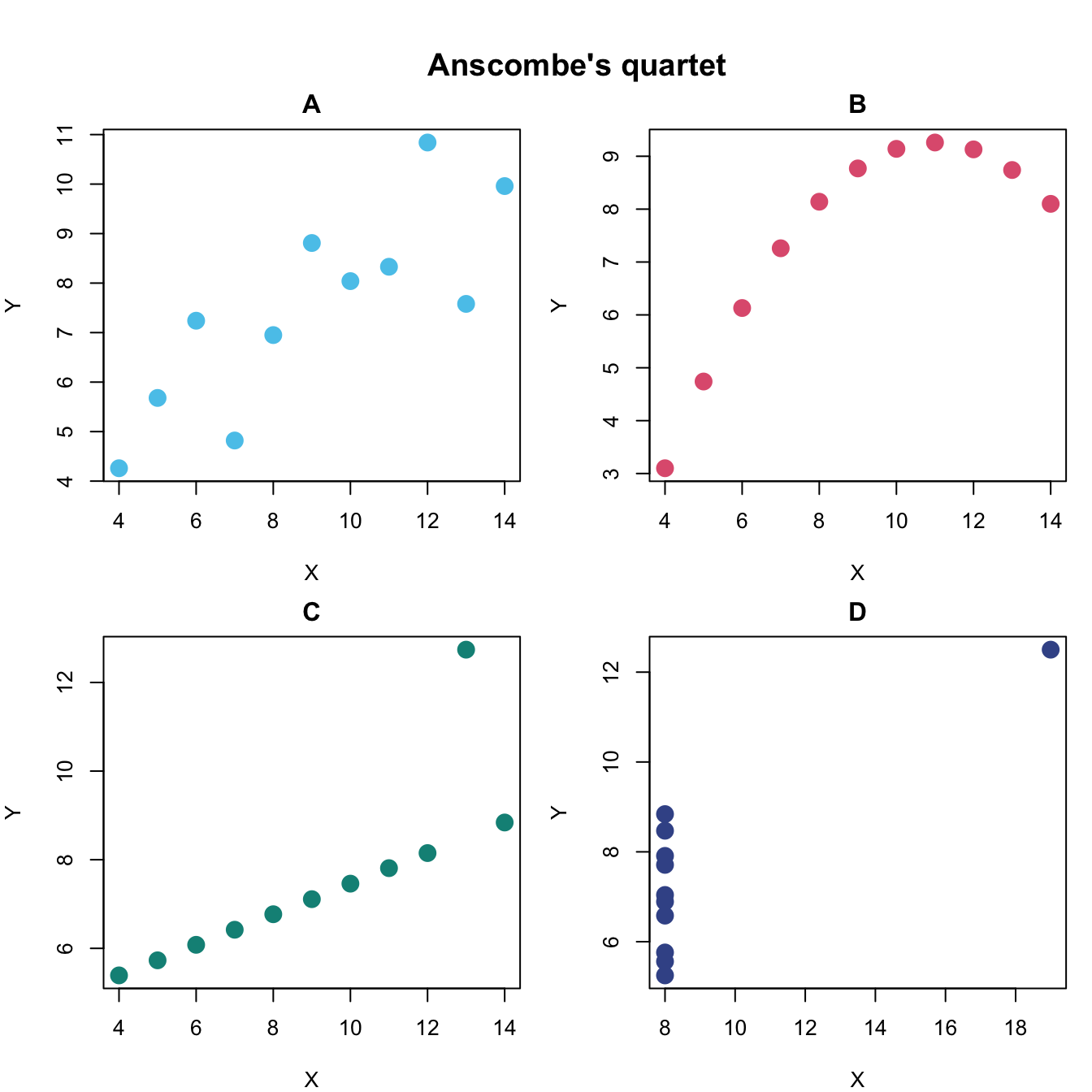Figure 3.6: Scatterplots of Anscombe’s quartet.

### 3.5.8 Bonus: More exercises

1. Re-create the Venn diagram from Chapter 1 (on the areas of data science).

2. Create a misleading and a transparent visualization for the same data.